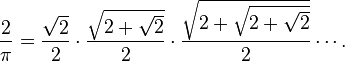## Viete’s Formula and Spinning Pizza

Have you seen Viete’s formula?:It’s a special case of a trig identity found by Euler:If you plug in$\pi/2$ to the trig identity and use the half-angle formula for cosine over and over, you get Viete’s formula.

But why would you want to consecutively cut angles in half and multiply their cosines? Well, you might be eating pizza.

You have a slice of pizza that is too hot to hold, so you want to balance it on your fork and gnaw at it instead. There’s a precise spot on the underside of the slice where the fork should go.This point is called the slice’s center of mass, and we’re going to find it. By symmetry, it must be on the line cutting the slice in half lengthwise, but we don’t yet know how far down. It depends on the shape of the slice, which we measure by$\theta$, the angle its edges make.The center of mass of the slice of pizza is a green dot. It lies on the line cutting the slice in half vertically.

A bigger slice will have its center of mass closer to the tip. We would like to know$r(\theta)$, the distance from the tip to the center of mass as a function of$\theta$.

There are two limiting cases – a very skinny slice and a whole pie. A very skinny slice is basically an isosceles triangle. Its center of mass is 2/3 the way from the tip to the edge1, so$\lim_{\theta \to 0} r(\theta) = \frac{2}{3}R$.

Let’s choose the radius of the pizza as unit of length, so$R = 1$ from here on.

In the other limiting case, an entire pizza has its center of mass right at the tip (i.e. center), so$r(2\pi) = 0$.

To investigate intermediate cases, we start with a slice of angle$\theta$ and imagine cutting it in half lengthwise, creating two skinny pieces of angle$\theta/2$. These have their own centers of mass at$r(\theta/2)$.

The center of mass of the big piece is on the line connecting the smaller pieces’ centers of mass.A bit of trigonometry tells us$r(\theta) = r(\theta/2)\cos(\theta/4)$

If we take this formula and divide all angles by$2$, we get a formula for$r(\theta/2)$. We substitute this for where$r(\theta/2)$ appeared in the original. We obtain$r(\theta) = \left[r(\theta/4)\cos(\theta/8)\right]\cos(\theta/4)$

Repeat the process ad infinitum. Rearranging the order of the terms and substituting the limiting value of$r$ for small$\theta$, we get$r(\theta) =\frac{2}{3} \cos(\theta/4)\cos(\theta/8)\cos(\theta/16)\ldots$

It involves one half of Euler’s trig identity. If we find$r(\theta)$ by a different method and get a different expression for it, we can set our two expressions for$r(\theta)$ equal to each other, and prove Euler’s identity. We’ll do this by invoking some physics ideas.

Suppose you’re spinning some pizza dough in the air. You know, like this:

If the pizza is spinning, each little bit of dough undergoes centripetal acceleration. Where there’s acceleration, there’s force. The pizza isn’t touching anything, so the force on any one piece of pizza must be coming from the rest of the pizza.

Let’s again examine a slice of size$\theta$, this time still attached to the spinning pizza. It has two forces of size$F$ acting on it; one force is exerted by the slice to its left and one by the slice to its right.There are two forces on the slice - one from the pizza to the left and one from the pizza to the right. They're both drawn originating from the center of mass. The slice is accelerating towards its tip (red arrow).

The sum of these forces is the mass of the slice times the acceleration of its center of mass. That acceleration is$\omega^2 r(\theta)$. Hence, if we determine the forces we can deduce$r(\theta)$.

Some trigonometry shows that the net force is$2F\sin(\theta/2)$.

Equating this to mass times acceleration, we get$2F\sin(\theta/2) = \frac{m \theta}{2\pi} \omega^2 r(\theta)$

We might as well let$m = \omega = 1$ and solve for$r$ to get$r(\theta) = 2F\sin(\theta/2)\frac{2 \pi}{\theta}$.

We still need to determine$F$, but we can do that because we know$r(\theta) \to 2/3$ as$\theta \to 0$. After a little algebra, we get$r(\theta) = \frac{4}{3} \frac{sin(\theta/2)}{\theta}$

This gives us the sought two expressions for$r$. We can now equate them and simplify to$\frac{sin(\theta)}{\theta} = \cos(\theta/2)\cos(\theta/4)\cos(\theta/8)\ldots$

1) To see why an isosceles triangle’s center of mass is 2/3 up the altitude, first show it’s true for an equilateral triangle. Then explain why all isosceles triangles have their center of mass the same fraction of the way down the altitude.